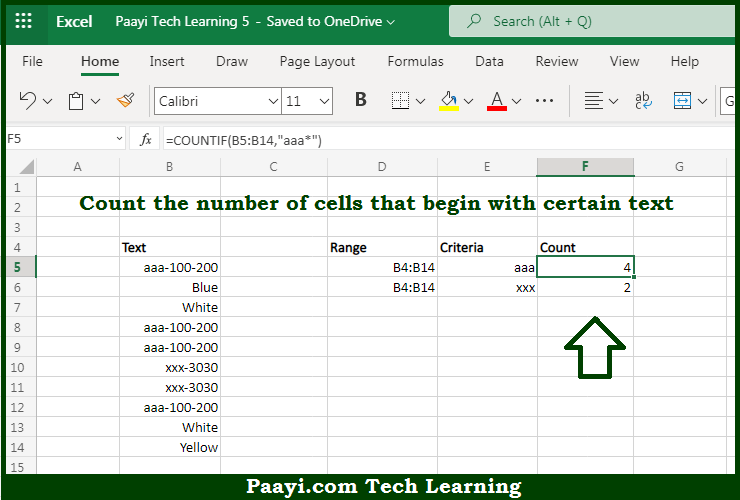# Learn How to Count Cells that Begin With Particular Text in Microsoft Excel

Written by | 0 Comments | 564 Views

In this article, you will learn how to COUNT various things in Microsoft Excel using a single or combination of functions and its purpose in Microsoft Excel. You will also get to know how to count cells that begin with particular text and see the generic

Count Cells that Begin With Particular Text in Microsoft Excel

The main purpose of this formula is to count cells that begin with a particular text. Here we will learn how to count cells that begin with the text string in Microsoft Excel.  That implies, with the help of a formula based on the  COUNTIF function you can able to count the number of cells that begin with certain text. It should be noted that the general formula "rng" is a range of cells, "txt" represents the text that cells should begin with, and "*" is a wildcard matching any number of characters. So, with the help of this formula, you can able to count cells begin with a particular text.

General Formula to COUNT Cells that Begin With Particular Text

=COUNTIF(rng,"txt*")

The Explanation for the COUNT Cells that Begin With Particular TextSo we know that with the help of the given formula above you can able to count cells that begin with a particular text. Here we will learn how to count cells that begin with the text string in Microsoft Excel. As we know that, the COUNTIF function is used to count the number of cells in the range that begin with txt by matching the content of each cell against the pattern "txt*", supplied as the criteria. The "*" symbol or the asterisk is a wildcard in Microsoft Excel. It should be noted that the count of cells that match this pattern is returned as a number. So, with the help of this formula, you can able to count cells that begin with a particular text.